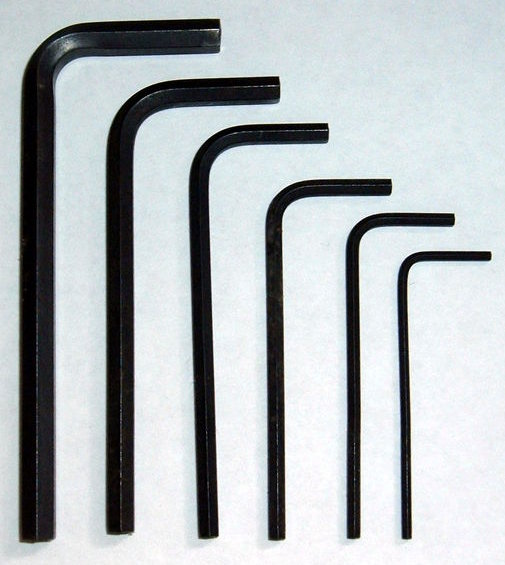Mechanics working on some car engines use common fractions.

Many drill bits, sockets and Allen keys are in Imperial sizes such as 12 inch, 14 inch, 18 inch and so on.

Steps to add and subtract common fractions are:

• Step 1 - find the common denominator (the number that both bottom numbers will divide into without a remainder)
• Step 2 - find the equivalent fractions
• Step 3 - add or subtract the equivalent fractions
• Step 4 - change to a mixed number or simplify if needed.

## Example One - Adding Allen KeysAllen keys are used to measure gaps in car engines. Into a gap, Allen keys with thicknesses of 12 inch and 14 inch can fit. What is the width of the gap?

12 + 14
= 24 + 14 (Steps 1 and 2)
= 34 (Step 3)

## Example Two - Adding Allen Keys

What is the width of a gap into which Allen keys with thicknesses of 38 inch and 116 inch can fit?

38 + 116
= 616 + 116 (Steps 1 and 2)
= 716 (Step 3)

## Example Three - Adding Pizza SlicesWhat is the total of 34 pizza and 23 pizza?

34 + 23
= 912 + 812 (Steps 1 and 2)
= 1712 (Step 3)
= 1 512 (Step 4)

## Example Four - Subtracting Pizza Slices

There is 34 of a pizza. If I eat 23 pizza, how much is left?

3423
= 912812 (Steps 1 and 2)
= 112 (Step 3)

Q1. 12 + 13
Q2. 34 + 13
Q3. 78 + 34
Q4. 5638

A1. 56
A2. 1 112
A3. 1 58
A4. 1124

## Example Five - Adding Mixed NumbersAdd 1 34 and 1 23.

Change these mixed numbers to improper fractions first.
1 34 + 1 23
= 74 + 53
= 2112 + 2012
= 4112
= 3 712

Q1. 2 18 + 3 34
Q2. 3 14 – 1 15

A1. 5 78
A2. 2 120

## Maths Fun

Bob loves to count. One day, his teacher put a pile of pencils on Bob's desk. Bob began to count the pencils. He told his teacher the following facts about the pencils:

• When I count the pencils by two, I have one left over.
• When I count the pencils by threes, I have one left over.
• When I count the pencils by fours, I have one left over.
• When I count them by sevens, I have none left over.

How many pencils are there?